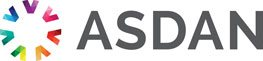# Lifeskills Challenge

## Working with probability

### Challenge reference: 3115

Notional learning hours 20 1 The learner will develop an understanding of probability and state the likely outcome in mathematical terms. Please log in to see the rest of this challenge

### Learning outcomes

What the learner needs to know, understand or be able to do

The learner will:

1. Understand the basic concepts and terminology associated with probability.

2. Be able to establish the probability of an event.

### Assessment criteria

What the learner need to demonstrate in order to meet the learning outcome

The learner can:

• Define probability
• Explain how the probability of an event is measured
• Describe how probability is determined
• Identify the likelihood of different events happening
• Collect data about a topic
• Display the data in an appropriate way
• Calculate the probability of an event
• Express the probability of the event